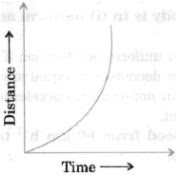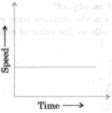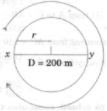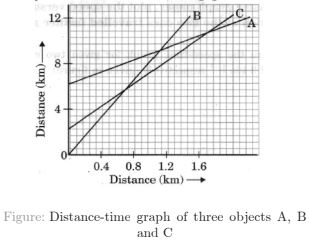# NCERT Solutions for Class 9 Science Chapter 8 Motion

 Chapter Name NCERT Solutions for Class 9 Science Chapter 8 Motion Topics Covered NCERT Intext QuestionsNCERT Exercise Solutions Related Study NCERT Solutions for Class 9 ScienceNCERT Revision Notes for Class 9 ScienceImportant Questions for Class 9 ScienceMCQ for Class 9 ScienceNCERT Exemplar Questions For Class 9 Science

### NCERT Intext Questions

1. An object has moved through a distance. Can it have zero displacement? If yes, support your answer with an example.

Solution

Yes, an object can have zero displacement provided that final position of the object coincides with its initial position.

For example: If a person moves from home to store and stands on place from where he started then here displacement will be zero.

2. A farmer moves along the boundary of a square field of side 10 m in 40 s. What will be the magnitude of displacement of the farmer at the end of 2 minutes 20 seconds from his initial position?

Solution

Given,
Side of the square field = 10 m
So, perimeter = 10 m × 4 = 40 m
Farmer moves along the boundary in 40 s.
Displacement after 2 m 20 s = 2 × 60 s + 20 s = 140 s = ?
Since in 40 s farmer moves 40 m.
Therefore, in 1 s distance covered by farmer = 40/40 m = 1 m
Therefore, in 140 s distance covered by farmer = 1 × 140 m = 140 m
Now, number of rotation of cover 140 along the boundary = (total distance/Perimeter)
= 140 m/40 m = 3.5 round
Thus, after 3.5 round farmer wil at point C of the field.
Therefore,
Thus, after 2 minutes 20 seconds the displacement of farmer will be equal to 14.14 m north east from initial position.

3. Which of the following is true for displacement?
(a) It cannot be zero.
(b) Its magnitude is greater than the distance travelled by the object.

Solution

None of the statement is true for displacement. First statement is false because displacement can be zero. Second statement is false as displacement is less than or equal to the distance travelled by the object.

4. Distinguish between speed and velocity.

Solution

Difference between Speed and Velocity

 Speed Velocity Speed is the distance travelled by an object in a given interval of time. Velocity is the displacement of an object in a given interval of time. Speed = distance/time Velocity = displacement/time Scalar quantity, i.e. it has only magnitude. Vector quantity, i.e. it has both magnitude as well as direction.

5. Under what condition(s) is the magnitude of average velocity of an object equal to its average speed ?

Solution

The magnitude of average velocity of an object is equal to its average speed when an object is moving in a straight line in a given direction only.

6. What does the odometer of an automobile measure ?

Solution

The odometer of an automobile measures the distance covered by an automobile in given time.

7. What does the path of an object look like when it is in uniform motion?

Solution

When an object having uniform motion, it moves along a straight line path.

8. During an experiment, a signal from a spaceship reached the ground station in five minutes. What was the distance of the spaceship from the ground station ? The signal travels at the speed of light, that is, 3 × 108  ms-1 .

Solution

Speed = 3 × 108 ms-1
Time = 5 min = 5 × 60 = 300 seconds
Distance = Speed × Time
Distance = 3 × 108 ms-1 × 300 seconds
= 9 × 1010 m

9. When will you say a body is in
(i) uniform acceleration?
(ii) non-uniform acceleration?

Solution

(i) A body is said to be in uniform acceleration if it travels in a straight line and its velocity increases or decreases by equal amounts in equal intervals of time.
(ii) A body is said to be in non-uniform acceleration if the rate of change of its velocity is not constant.

10. A bus decreases its speed from 80 km h-1 to 60 km h-1. in 5 s. Find the acceleration of the bus.

Solution

Initial speed of the bus,
u = 80 km/h = 80 × (5/18) = 22.2 m/s
= 60 × (5/18) = 16.6 m/s
Final speed of the bus,
v = 60 km/h
Time taken to decrease the speed, t = 5 s
Acceleration,
a = (v – u)/t = (16.66 – 22.22)/5 = - 1.112 m/s2

11. A train starting from a railway station and moving with uniform acceleration attains a speed 40 km h-1 in 10 minutes. Find its acceleration.

Solution

Initial velocity of the train, u = 0
Final velocity of the train,
v = 40 km/h = 40 × (5/18) = 11.11 m/s
Time taken,
t = 10 min = 10 × 60 = 600 s
Acceleration,
a = (v- u)/t = (11.11 -0)/600 = 0.0185 m/s2
Hence, the acceleration of the train is 0.0185 m/s2

12. What is the nature of the distance-time graphs for uniform and non-uniform motion of an object?

Solution

When the motion is uniform, the distance – time graph is a straight line having a definite slope. The slope tells us the value of constant velocity of the object.

When the motion is non – uniform, the distance time graph is a curve whose slope varies from point to point.13. What can you say about the motion of an object whose distance-time graph is a straight line parallel to the time axis?

Solution

If distance-time graph is a straight line parallel to the time axis, the body is at rest and the distance of the object does not change with time.14. What can you say about the motion of an object if its speed-time graph is a straight line parallel to the time axis?

Solution

If speed-time graph is a straight line parallel to the time axis, the object is moving uniformly.15. What is the quantity which is measured by the area occupied below the velocity-time graph?

Solution

Area occupied below the velocity-time graph of an object gives the magnitude of displacement or the distance covered during the given time interval.

16. A bus starting from rest moves with a uniform acceleration of 0.1 ms–2 for 2 minutes. Find (a) the speed acquired, (b) the distance travelled.

Solution

Initial speed of the bus, u = 0
Acceleration, a = 0.1 m/s2
Time taken, t = 2 minutes = 120 s
(a) v = u + at
⇒ v = 0 + 0.1 × 120
⇒ v = 12 ms–1
(b) According to the third equation of motion :
v2 – u2 = 2 as
where, s is the distance covered by the bus
(12)2 – (0)2 = 2(0.1) s
⇒ s = 720 m
Speed acquired by the bus is 12 m/s.
Distance travelled by the bus is 720 m.

17. A train is travelling at a speed of 90 km h–1. Brakes are applied so as to produce a uniform acceleration of – 0.5 m s–2. Find how far the train will go before it is brought to rest.

Solution

Initial speed of the train,
u = 90 km/h = 25 m/s
Final speed of the train,
v = 0 (finally the train comes to rest)
Acceleration = – 0.5 m
According to third equation of motion :
v2 = u2 + 2 as
⇒ (0)2 = (25)2 + 2 (– 0.5) s
Where, s is the distance covered by the train
s = 252/2(0.5) = 625 m
The train will cover a distance of 625 m before it comes to rest.

18. A trolley, while going down an inclined plane, has an acceleration of 2 cm s–2. What will be its velocity 3 s after the start?

Solution

Initial velocity of trolley, u = 0 cm s–1
Acceleration, a = 2 cm s–2
Time, t = 3 s
We know that final velocity,
v = u + at
= 0 + 2 × 3 cm s–1
Therefore, the velocity of train after 3 seconds = 6 cm s–1

19. A racing car has a uniform acceleration of 4 ms–2. What distance will it cover in 10 s after start?

Solution

Initial velocity of the car, u = 0 ms–1
Acceleration, a = 4 m s–2
Time, t = 10 s
We know, distance, s = ut + (1/2)at2
Therefore, distance covered by car in 10 seconds
= 0 × 10 + (1/2) × 4 × 102
= 0 + (1/2) × 4 × 10 × 10 m
= (1/2) × 400 m = 200 m

20. A stone is thrown in a vertically upward direction with a velocity of 5 m s–1. If the acceleration of the stone during its motion is 10 m s–2 in the downward direction, what will be the height attained by the stone and how much time will it take to reach there?

Solution

Given initial velocity of stone, u= 5 m s–1
Terminal velocity of stone, (v) = 0 ms-1 (at maximum height)
Downward of negative acceleration, a = –10 m s–2
(acceleration is taken negative because it is in downward direction, i.e. the direction of acceleration is opposite to that of velocity.
We know that 2 as = v2 – u2
Therefore, height attained by the stone,
s = (v2 – u2)/2a
⇒ s = (02 - 52)/2 × -10 = (-25/-20)m = 1.25 m
Also we know that final velocity,
v = u + at
or, Time, t = (v – u)/a
Therefore, time, t taken by stone to attain the height,
s = (0 – 5)/-10 s = 0.5

### NCERT Exercises Solutions

1. An athlete completes one round of a circular track of diameter 200 m in 40 s. What will be the distance covered and the displacement at the end of 2 minutes 20 s?

Solution

Diameter of circular track, D = 200 m
Radius of circular track,r = 200/2 = 100 m
Time taken by the athlete for one round (t) = 40 s
Distance covered by athlete in one round
s = 2πr = 2 × 22/7 × 100
Speed of the athlete
v = Distance/Time = (2×2200)/(7×40) = 4400/(7× 40)
Therefore, distance covered in 140 s
= Speed (s) × Time(t)
= 4400/(7×40) ×(2×60 + 20)
= 4400/(7×40)×140
= (4400 ×140)/(7×40) = 2200 m
Number of round in 40 s =1 round
Number of round in 140 s = 140/40 = 7/2
After taking start from position X, the athlete will be at position Y after 7/2 rounds as shown in figureHence, displacement of the athlete with respect to initial position at x = xy
= Diameter of circular track
= 200 m

2. Joseph jogs from one end A to the other end B of a straight 300 m road in 2 minutes 30 seconds and then turns around and jogs 100 m back to point C in another 1 minute. What are Joseph’s average speeds and velocities in jogging (a) from A to B and (b) from A to C?

Solution

Total distance covered from AB = 300 m
Total time taken = 2× 60 + 30 s = 150 sTherefore, average speed from AB
= (Total Distance/Total Time) = 300/150 ms-1 = 2 ms-1
Therefore, velocity from AB
= Displacement AB/Time
= 300/150 ms-1 = 2 ms-1
Total distance covered from AC
= AB + BC = 300 + 200 m
Total time taken from A to C
= Time take for AB + Time taken for BC
= (2× 60 + 30) + 60 s = 210 s
Therefore, average speed from AC = Total distance/total time
= 400/210 ms-1
= 1.904 ms-1
Displacement (s) from A to C
= AB – BC
= 300 – 100 m = 200 m
Time (t) taken for displacement from AC = 210 s
Therefore, velocity from AC
= Displacement(s)/Time(t) = 200/ 210 ms-1 = 0.952 ms-1

3. Q. a. Abdul, while driving to school, computes the speed for his trip to be 20 km h–1. On his return trip along the same route, there is less traffic and the average speed is 30 km h–1. What is the average speed for Abdul’s trip?

Solution

Let the distance of Abdul’s school from his residence be s km .
As during his trip from his residence to school, his average speed,
V1 = 20 km h-1
Hence, in covering a distance s km, he takes the time
t1 = s/V1 = sh/20
Similarly, during the return trip, he takes the time
t2 = s/V2 = sh/30
Average speed for Abdul’s entire trip,4. A motorboat starting from rest on a lake accelerates in a straight line at a constant rate of 3.0 m s–2 for 8.0 s. How far does the boat travel during this time?

Solution

Given initial velocity of motorboat, u = 0
Acceleration of motorboat, a = 3.0 m s–2
Time under consideration, t = 8.0 s
We know that distance, s = ut + (1/2)at2
Therefore, the distance travel by motorboat
= 0 × 8 + (1/2)3.0 × 82
= (1/2) × 3 × 8 × 8 m = 96 m

5. A driver of a car travelling at 52 km h–1 applies the brakes and accelerates uniformly in the opposite direction. The car stops in 5 s. Another driver going at 3 km h–1 in another car applies his brakes slowly and stops in 10 s. On the same graph paper, plot the speed versus time graphs for the two cars. Which of the two cars travelled farther after the brakes were applied?

Solution

PR and SQ are the speed-time graph for given two cars with initial speeds 52 km h–1 and 3 km h–1 respectively (given in figure).6. Figure slows the distance – time graph of three objects A, B and C. Study the graph and Answer the following questions :(a) Which of the three is travelling the fastest ?
(b) Are all three ever at the same point on the road ?
(c) How far has C travelled when B passes A ?
(d) How far has B travelled by the time it passes C ?

Solution

(a) Speed = Distance/Time
Slope of graph = Y – axis /X – axis = Distance/Time
Therefore, speed = slope of the graph
Since slope of object B is greater than objects A and C, it is travelling the fastest.
(b) All three objects A, B and C never meet at a single point. Thus, they were never at the same point on road.
(c)7 square box = 4 km
1 square box = 4/7 km
C is 4 blocks away from origin, therefore, initial distance of C from origin = 16/7 km
Distance of C from origin when B passes A = 8 km
Thus, distance travelled by C when B passes A
= 8 – (16/7) = (56 – 16)/7 = 40/7 = 5.714 km
(d) Distance travelled by B by the time it passes C = 9 square boxes
9 × (4/7) = 36/7 = 5.143 km

7. A ball is gently dropped from a height of 20 m. If its velocity increases uniformly at the rate of 10 m s–2, with what velocity will it strike the ground? After what time will it strike the ground?

Solution

Let us assume, the final velocity with which ball will strike the ground be and time it takes to strike the ground be t. Initial velocity of ball, u = 0
Distance or height of fall, s = 20 m
Downward acceleration, a = 10 m s–2
As we know, 2as = v2 – u2
v2 = 2as + u2
= 2 × 10 × 20 + 0 = 400
Final velocity of ball,
v = 20 ms-1
t = (v – u)/a
Time taken by the ball to strike = (20 – 0)10 = 20/10 seconds

8. The speed-time graph for a car is shown in figure.(a) Find out how far the car travels in the first 4 seconds. Shade the area on the graph that represents the distance travelled by the car during the period.
(b) Which part of the graph represents uniform motion of the car?

Solution

(a)The shaded area which is equal to ½ × 4 × 6 = 12 m represents the distance travelled by the car in the first 4 s.
(b)

The part of the graph between time 6 s to 10 s represents uniform motion of the car.

9. State which of the following situations are possible and give an example for each or these :
(a) an object with a constant acceleration but with zero velocity.
(b) an object moving in a certain direction with an acceleration in the perpendicular direction.

Solution

(a) Possible a ball has zero velocity when a ball is thrown up at maximum height. It will have constant acceleration due to gravity, which is equal to 9.8 m/s2 .
(b) Possible a car’s acceleration is perpendicular to its direction when a car is moving in a circular track.

10. An artificial satellite is moving in a circular orbit of radius 42250 km. Calculate its speed if it takes 24 hours to revolve around the Earth.

Solution

Radius of the circular orbit, r = 42250 km

Time taken to revolve around the Earth, t = 24 h